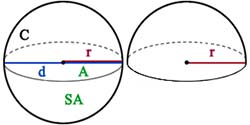# Sphere Calculator

The Sphere Calculator allows you to calculate three key properties of a sphere: area, volume, and circumference.

Circle CalculatorSphere Calculator

sq. units

cu. units

Hemisphere Calculator

0 square units

0 cubic units

## Sphere Calculations

The calculator uses the following formulas to compute the volume, circumference, and area of a sphere:

• Volume of a sphere: V = ( 4/3 )πr3
• Circumference of a sphere: C = 2πr
• Surface Area of a sphere: SA = 4πr2

## Hemisphere Calculations

A hemisphere is half of a sphere. The calculator can estimate the volume and surface area of a hemisphere using the following formulas:

• Volume of a hemisphere: V = ( ( 4/3 )πr3 ) / 2
• Surface Area of a hemisphere: A = ( 4πr2 / 2 ) + πr2 = 3πr2
• Where π is the constant (3.141592654)

To calculate the surface area of a hemisphere, the calculator first computes the surface area of a full sphere and divides it by two. The flat end of the hemisphere is then added to this value using the area calculations of a circle. The resulting sum gives the total surface area of the hemisphere.